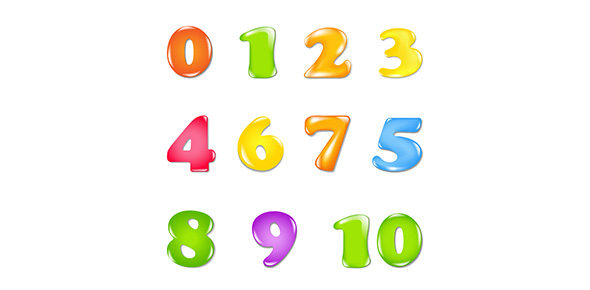# Reduction And Oxidation Reactions

6 Questions | Total Attempts: 151SettingsThis quiz will test you on the material given to you in the two Wikipedia articles and the YouTube video.

• 1.
In a reduction reaction, the element gains electrons.
• A.

True

• B.

False

• 2.
Sulfuric acid is a weak acid.
• A.

True

• B.

False

• 3.
Consider the dehydration of sugar by sulfuric acid. C12H22O11 (Sugar) + H2SO4 (Sulfuric Acid) → 12C (Graphite) +11H2O (Water) + H2SO4+ Heat Is this a redox reaction?
• A.

Yes. Carbon is oxidized and Oxygen is reduced.

• B.

Yes. Carbon is reduced and Oxygen is oxidized.

• C.

Yes. Carbon is oxidized and Sulfur is reduced

• D.

No. This is not a redox reaction.

• 4.
The reaction in the second video can be described by the following reaction.Fe(s) +CuSO4(aq)  ---> FeSO4(aq) + Cu(s)What are the oxidation states of the reactants?
• A.

Fe =0, Cu = +2, S = +6, O = -2

• B.

Fe =+2, Cu = -2, S = +6, O = -2

• C.

Fe =+3, Cu = +2, S = +6, O = -1

• D.

Fe =0, Cu = +2, S = -6, O = +2

• 5.
What are the oxidation states of the products in the reaction Fe(s) +CuSO4(aq)  ---> FeSO4(aq) + Cu(s)
• A.

Fe = -1, S = +6, O =+2, Cu = +2

• B.

Fe = +1, S = -6, O = -1, Cu = -1

• C.

Fe = +2, S = +6, O = -2, Cu = 0

• D.

Fe = 0, S = +2, O = +6, Cu = +1

• 6.
Is the reaction Fe(s) +CuSO4(aq)  ---> FeSO4(aq) + Cu(s) a redox reaction?
• A.

True

• B.

False

Related TopicsBack to top